# LOG#073. The G2 system.

The second paper I am going to discuss today is this one:

http://inspirehep.net/record/844954?ln=en

In Note on the natural system of units, Sudarshan, Boya and Rivera introduce a new kind of “fundamental system of units”, that we could call G2 system  or the Boya-Rivera-Sudarshan system (BRS system for short). After a summary of the Gamov-Ivanenko-Landau-Okun cube (GILO cube) and the Planck natural units, they make the following question:

Can we change the gravitational constant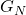for something else?

They ask this question due to the fact theseems to be a little different from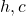. Indeed, many researchers in quantum gravity use to changewith the Planck length as fundamental unit! The G2 system proposal is based in some kind of two dimensional (2d) world. Sudarshan, Boya and Rivera search for a “new constant”such assubstitutes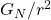in the Newton’s gravitational law.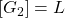in this new “partial” fundamental system. Therefore, we haveand the physical dimensions of time, length and mass are expressed in terms ofas follows (we could useinstead of h, that is not essential here as we do know from previous discussions) :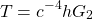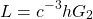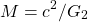In fact, they remark that sincederives from a 2+1 dimensional world and Einstein Field equations are generally “trivial” in 2+1 spacetime,, surprisingly, is not related to gravitation at all! We are almost “free” to fixwith some alternative procedure. As we wish to base the G2 system in well known physics, the election they do foris the trivial one ( however I am yet thinking about what we could obtain with some non-trivial alternative definition of):and any other equivalent expression to it. Please, note that if we fix the Planck length to unit, we get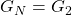, so it is equivalent to speak aboutorin a system of units where Planck length is set to the unit. However, the proposal is independent of this fact, since, as we said above, we could choose some other non-trivial definition for, although I don’t know what kind of guide we could follow in those alternative and non-trivial definition.

The final remark I would like to make here is that, whatever we choose instead of, it is ESSENTIAL to a quantum theory of gravity, provided it exists, it works and it is “clear” from its foundational principles.

See you in my next blog post!

This site uses Akismet to reduce spam. Learn how your comment data is processed.